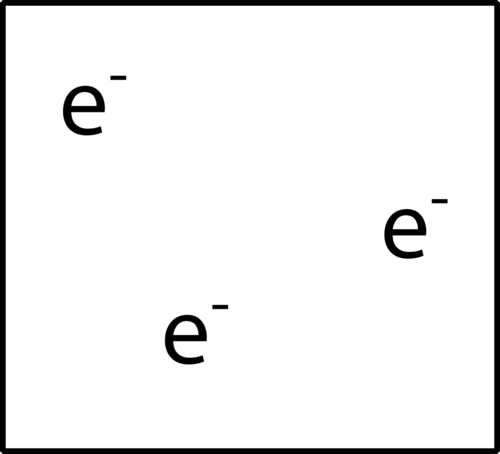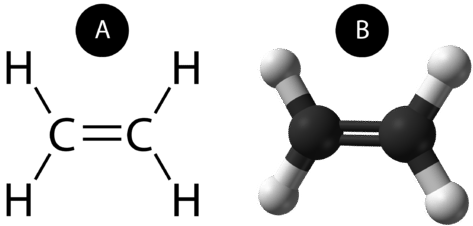# 9.7: Multiple Covalent Bonds

$$\newcommand{\vecs}{\overset { \rightharpoonup} {\mathbf{#1}} }$$ $$\newcommand{\vecd}{\overset{-\!-\!\rightharpoonup}{\vphantom{a}\smash {#1}}}$$$$\newcommand{\id}{\mathrm{id}}$$ $$\newcommand{\Span}{\mathrm{span}}$$ $$\newcommand{\kernel}{\mathrm{null}\,}$$ $$\newcommand{\range}{\mathrm{range}\,}$$ $$\newcommand{\RealPart}{\mathrm{Re}}$$ $$\newcommand{\ImaginaryPart}{\mathrm{Im}}$$ $$\newcommand{\Argument}{\mathrm{Arg}}$$ $$\newcommand{\norm}{\| #1 \|}$$ $$\newcommand{\inner}{\langle #1, #2 \rangle}$$ $$\newcommand{\Span}{\mathrm{span}}$$ $$\newcommand{\id}{\mathrm{id}}$$ $$\newcommand{\Span}{\mathrm{span}}$$ $$\newcommand{\kernel}{\mathrm{null}\,}$$ $$\newcommand{\range}{\mathrm{range}\,}$$ $$\newcommand{\RealPart}{\mathrm{Re}}$$ $$\newcommand{\ImaginaryPart}{\mathrm{Im}}$$ $$\newcommand{\Argument}{\mathrm{Arg}}$$ $$\newcommand{\norm}{\| #1 \|}$$ $$\newcommand{\inner}{\langle #1, #2 \rangle}$$ $$\newcommand{\Span}{\mathrm{span}}$$Figure $$\PageIndex{1}$$ (Credit: Joy Sheng; Source: CK-12 Foundation; License: CC BY-NC 3.0)

### What do you do with your leftovers?

When working with covalent structures, it sometimes looks like there are leftover electrons. Maybe you have applied the rules you learned so far, but there are still some electrons hanging out there, unattached. You can't just leave them there...So, where do you put them?

## Multiple Covalent Bonds

Some molecules are not able to satisfy the octet rule by making only single covalent bonds between the atoms. Consider the compound ethene, which has a molecular formula of $$\ce{C_2H_4}$$. The carbon atoms are bonded together, with each carbon also bonded to two hydrogen atoms.

two $$\ce{C}$$ atoms $$= 2 \times 4 = 8$$ valence electrons

four $$\ce{H}$$ atoms $$= 4 \times 1 = 4$$ valence electrons

= total of 12 valence electrons in the molecule

If the Lewis electron dot structure was drawn with a single bond between the carbon atoms and with the octet rule followed, it would look like this:Figure $$\PageIndex{2}$$: Incorrect dot structure of ethene. (Credit: Joy Sheng; Source: CK-12 Foundation; License: CC BY-NC 3.0)

This Lewis structure is incorrect because it contains a total of 14 electrons. However, the Lewis structure can be changed by eliminating the lone pairs on the carbon atoms and having the carbon atoms share two pairs, instead of only one pair, of electrons.Figure $$\PageIndex{3}$$: Correct dot structure for ethene. (Credit: Joy Sheng; Source: CK-12 Foundation; License: CC BY-NC 3.0)

A double covalent bond is a covalent bond formed by atoms that share two pairs of electrons. The double covalent bond that occurs between the two carbon atoms in ethane can also be represented by a structural formula and with a molecular model, as shown in the figure below.Figure $$\PageIndex{4}$$: (A) The structural model for $$\ce{C_2H_4}$$ consists of a double covalent bond between the two carbon atoms and single bonds to the hydrogen atoms. (B) Molecular model of $$\ce{C_2H_4}$$. (Credit: Ben Mills (Wikimedia: Benjah-bmm27); Source: (A) http://commons.wikimedia.org/wiki/File:Ethylene-CRC-MW-dimensions-2D-Vector.svg; (B) http://commons.wikimedia.org/wiki/File:Ethylene-CRC-MW-3D-balls.png; License: Public Domain)

A triple covalent bond is a covalent bond formed by atoms that share three pairs of electrons. The element nitrogen is a gas that composes the majority of Earth's atmosphere. A nitrogen atom has five valence electrons, which can be shown as one pair and three single electrons. When combining with another nitrogen atom to form a diatomic molecule, the three single electrons on each atom combine to form three shared pairs of electrons.Figure $$\PageIndex{5}$$: Triple bond in $$\ce{N_2}$$. (Credit: CK-12 Foundation - Joy Sheng, using 3D molecular structure by Ben Mills (Wikimedia: Benjah-bmm27); Source: Molecular structure: http://commons.wikimedia.org/wiki/File:Nitrogen-3D-vdW.png; License: CC BY-NC-SA 3.0; Molecule Public Domain)

Each nitrogen atom follows the octet rule with one lone pair of electrons, and six electrons that are shared between the atoms.

## Summary

• Lewis structures can be drawn for molecules that share multiple pairs of electrons.
• A double covalent bond is a covalent bond formed by atoms that share two pairs of electrons.
• A triple covalent bond is a covalent bond formed by atoms that share three pairs of electrons.

## Review

1. Why is the first ethene Lewis structure incorrect?
2. What do the single electrons in nitrogen do to form a triple bond?
3. Draw the Lewis structure for ethyne C2H2.

9.7: Multiple Covalent Bonds is shared under a CK-12 license and was authored, remixed, and/or curated by CK-12 Foundation via source content that was edited to conform to the style and standards of the LibreTexts platform; a detailed edit history is available upon request.